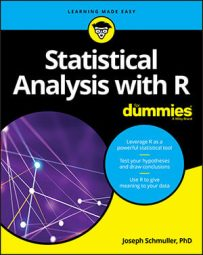##### Statistical Analysis with R For DummiesThe Base R graphics toolset will get you started, but if you really want to shine at visualization, it's a good idea to learn ggplot2. In ggplot2 is an easy-to-learn structure for R graphics code. To learn that structure, make sure you have ggplot2 in the library so that you can follow what comes next. (Find ggplot2 on the Packages tab and click its check box.)

A graph starts with `ggplot()`, which takes two arguments. The first argument is the source of the data. The second argument maps the data components of interest into components of the graph. The function that does the job is `aes()`.

To begin a histogram for `Price `in `Cars93`, the function is

```> ggplot(Cars93, aes(x=Price)) ``` The `aes() `function associates `Price `with the x-axis. In ggplot-world, this is called an aesthetic mapping. In fact, each argument to `aes() `is called an aesthetic.

This line of code draws the following figure, which is just a grid with a gray background and `Price `on the x-axis.

Well, what about the y-axis? Does anything in the data map into it? No. That's because this is a histogram and nothing explicitly in the data provides a y-value for each x. So you can't say "y=" in `aes()`. Instead, you let R do the work to calculate the heights of the bars in the histogram.

And what about that histogram? How do you put it into this blank grid? You have to add something indicating that you want to plot a histogram and let R take care of the rest. What you add is a `geom `function ("geom" is short for "geometric object").

These `geom `functions come in a variety of types. ggplot2 supplies one for almost every graphing need, and provides the flexibility to work with special cases. To draw a histogram, the `geom `function to use is called `geom_histogram()`.

How do you add `geom_histogram()` to `ggplot()`? With a plus sign:

`ggplot(Cars93, aes(x=Price)) +`

```geom_histogram() ``` This produces the following figure. The grammar rules tell ggplot2 that when the geometric object is a histogram, R does the necessary calculations on the data and produces the appropriate plot.

At the bare minimum, ggplot2 graphics code has to have data, aesthetic mappings, and a geometric object. It's like answering a logical sequence of questions: What's the source of the data? What parts of the data are you interested in? Which parts of the data correspond to which parts of the graph? How do you want the graph to look?

Beyond those minimum requirements, you can modify the graph. Each bar is called a bin, and by default, `ggplot() `uses 30 of them. After plotting the histogram, `ggplot() `displays an onscreen message that advises experimenting with binwidth (which, unsurprisingly, specifies the width of each bin) to change the graph's appearance. Accordingly, you use `binwidth = 5 `as an argument in `geom_histogram()`.

Additional arguments modify the way the bars look:

```geom_histogram(binwidth=5, color = "black", fill = "white") ``` With another function, `labs()`, you modify the labels for the axes and supply a title for the graph:

```labs(x = "Price (x \$1000)", y="Frequency",title="Prices of 93 Models of 1993 Cars")```

Altogether now:

```ggplot(Cars93, aes(x=Price)) + ``` ```geom_histogram(binwidth=5,color="black",fill="white") + ``` ```labs(x = "Price (x \$1000)", y="Frequency", title="Prices of 93 ``````Models of 1993 Cars") ``` The result is the following figure.